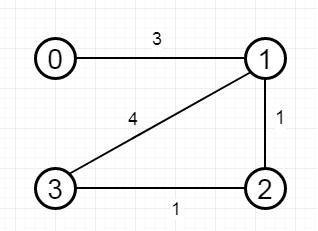# Find the City With the Smallest Number of Neighbors at a Threshold Distance in C++

Suppose there are n cities numbered from 0 to n-1. If we have the array edges where edges[i] = [fromi, toi, weighti] represents a bidirectional and weighted edge between cities fromi and toi, and given the integer distance threshold. We have to find the city with the smallest number of cities that are reachable through some path and whose distance is at most distance threshold, If there are more than one such cities, return the city with the greatest number.

So if the input is like −n is 4 and the distance threshold is also 4, then the output will be 3. This is because −

The neighboring cities at a distance threshold = 4 for each city are −

C0 -> [C1, C2]
C1 -> [C0, C2, C3]
C2 -> [C0, C1, C3]
C3 -> [C1, C2]

Cities 0 and 3 have 2 neighboring cities at a distance threshold = 4, but we have to return city 3 since it has the greatest number.

To solve this, we will follow these steps −

• Define a square matrix of order n x n called dp and fill this with infinity

• generate the adjacency matrix (cost matrix) of the graph and store into dp

• ret := 0 and cnt := infinity

• for k in range 0 to n – 1

• for i in range 0 to n – 1

• for j in range 0 to n – 1

• if i = j, then go for next iteration

• if dp[i, j] > dp[i, k] + dp[k, j], then

• dp[j, i] := dp[i, k] + dp[k, j]

• dp[i, j] := dp[i, k] + dp[k, j]

• for i in range 0 to n - 1

• temp := 0

• for j in range 0 to n – 1

• temp := temp + 1 when dp[i, j] <= t, otherwise temp remains same

• if temp <= cnt, then cnt := temp and ret := i

• return ret

## Example (C++)

Let us see the following implementation to get better understanding −

Live Demo

#include <bits/stdc++.h>
using namespace std;
class Solution {
public:
int findTheCity(int n, vector<vector<int>>& e, int t) {
vector < vector <int> > dp(n, vector <int>(n, 1e7));
for(int i = 0; i < e.size(); i++){
int u = e[i];
int v = e[i];
int w = e[i];
dp[u][v] = w;
dp[v][u] = w;
}
int ret = 0;
int cnt = INT_MAX;
for(int k = 0; k < n; k++){
for(int i = 0; i < n; i++){
for(int j = 0; j < n; j++){
if(i == j) continue;
if(dp[i][j] > dp[i][k] + dp[k][j]){
dp[j][i] = dp[i][j] = (dp[i][k] + dp[k][j]);
}
}
}
}
for(int i = 0; i < n; i++){
int temp = 0;
for(int j = 0; j < n; j++){
temp += (dp[i][j] <= t);
}
if(temp <= cnt){
cnt = temp;
ret = i;
}
}
return ret;
}
};
main(){
vector<vector<int>> v = {{0,1,3},{1,2,1},{1,3,4},{2,3,1}};
Solution ob;
cout << (ob.findTheCity(4, v, 4));
}

## Input

4
[[0,1,3],[1,2,1],[1,3,4],[2,3,1]]
4

## Output

3